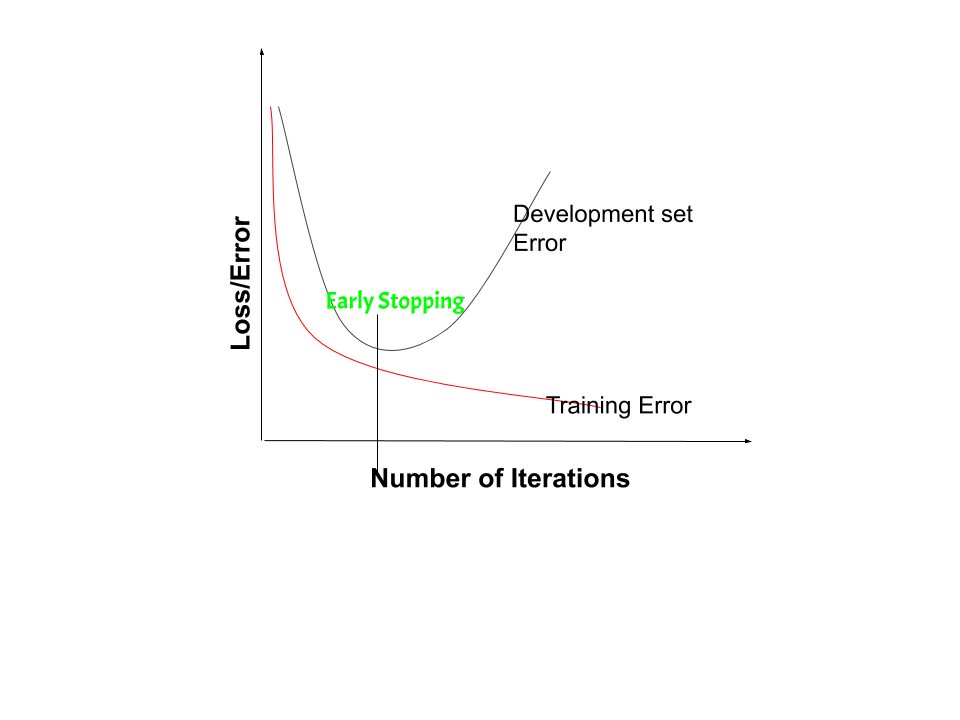# Regularization by Early Stopping

• Last Updated : 24 Oct, 2020

Regularization is a kind of regression where the learning algorithms are modified to reduce overfitting. This may incur a higher bias but will lead to lower variance when compared to non-regularized models i.e. increases generalization of the training algorithm.

In a general learning algorithm, the dataset is divided as a training set and test set. After each epoch of the algorithm, the parameters are updated accordingly after understanding the dataset. Finally, this trained model is applied to the test set. Generally, the training set error will be less compared to the test set error. This is because of overfitting whereby the algorithm memorizes the training data and produces the right results on the training set. So the model becomes highly exclusive to the training set and fails to produce accurate results for other datasets including the test set. Regularization techniques are used in such situations to reduce overfitting and increase the performance of the model on any general dataset. Early stopping is a popular regularization technique due to its simplicity and effectiveness.

Attention reader! Don’t stop learning now. Get hold of all the important Machine Learning Concepts with the Machine Learning Foundation Course at a student-friendly price and become industry ready.

Regularization by early stopping can be done either by dividing the dataset into training and test sets and then using cross-validation on the training set or by dividing the dataset into training, validation and test sets, in which case cross-validation is not required. Here, the second case is analyzed. In early stopping, the algorithm is trained using the training set and the point at which to stop training is determined from the validation set. Training error and validation error are analysed. The training error steadily decreases while validation error decreases until a point, after which it increases. This is because, during training, the learning model starts to overfit to the training data. This causes the training error to decrease while the validation error increases. So a model with better validation set error can be obtained if the parameters that give the least validation set error are used. Each time the error on the validation set decreases, a copy of the model parameters is stored. When the training algorithm terminates, these parameters which give the least validation set error are finally returned and not the last modified parameters.In Regularization by Early Stopping, we stop training the model when the performance of the model on the validation set is getting worse-increasing loss or decreasing accuracy or poorer values of the scoring metric. By plotting the error on the training dataset and the validation dataset together, both the errors decrease with a number of iterations until the point where the model starts to overfit. After this point, the training error still decreases but the validation error increases. So, even if training is continued after this point, early stopping essentially returns the set of parameters which were used at this point and so is equivalent to stopping training at that point. So, the final parameters returned will enable the model to have low variance and better generalization. The model at the time the training is stopped will have a better generalization performance than the model with the least training error. Early stopping can be thought of as implicit regularization, contrary to regularization via weight decay. This method is also efficient since it requires less amount of training data, which is not always available. Due to this fact, early stopping requires lesser time for training compared to other regularization methods. Repeating the early stopping process many times may result in the model overfitting the validation dataset, just as similar as overfitting occurs in the case of training data.

The number of iterations taken to train the model can be considered as a hyperparameter. Then the model has to find an optimum value for this hyperparameter(by hyperparameter tuning)for the best performance of the learning model.

My Personal Notes arrow_drop_up